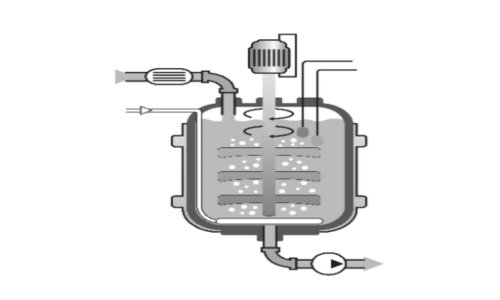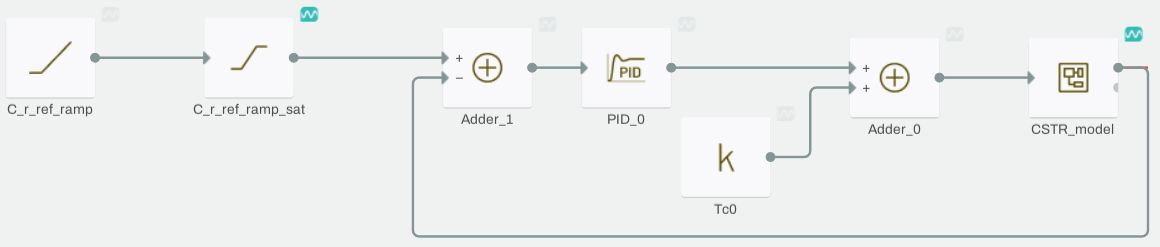# Designing a Continuous Stirred-Tank Reactor (CSTR)## CSTR Definition

A Continuous Stirred-Tank Reactor (CSTR) is a type of chemical reactor that has a wealth of applications in industrial processes. The CSTR models an irreversible chemical reaction which transforms a chemical liquid $$A$$ into a mix of chemicals. In this tutorial, we will investigate how to model this process in Collimator.

## Modeling the CSTR design equation

The CSTR can be modeled as a nonlinear system of differential equations:

$$\frac{d C_r}{dt} = \frac{F}{V}\left( C_{Ai}\left(t\right) - C_{r}\left(t\right) \right) -k_0 e^{\frac{-E}{R T_r\left(t\right)}} C_r\left(t\right)$$

$$\frac{d T_r\left(t\right)}{dt} = \frac{F}{V}\left( T_{Ai}\left(t\right) - T_{r}\left(t\right) \right)-\frac{\Delta H}{\rho C_p} k_0 e^{\frac{-E}{R T_r\left(t\right)}}-\frac{U A}{\rho C_p V}\left( T_{r}\left(t\right) - T_{c}\left(t\right) \right)$$

The CSTR variables and parameters are denoted in the following tables:

\begin{array} {|l|l|} \hline Variable & Unit & Description \\ \hline C_{Ai} & \frac{kmol}{m^3} & \text{Concentration of A in inlet flow} \\ \hline C_{r} & \frac{kmol}{m^3} & \text{Concentration of A in reactor mix} \\ \hline T_{Ai} & K  & \text{Temperature of inlet flow}\\ \hline T_{r} & K & \text{Temperature of reactor mix} \\ \hline T_{c} & K & \text{Temperature of cooling medium} \\ \hline  \end{array}

\begin{array} {|l|l|}\hline Parameter & Value & Unit & Description \\ \hline F & 1.2 & \frac{m^3}{h} & \text{Inlet flow rate}\\ \hline V & 1.2 & m^3 & \text{Reactor mix volume}\\ \hline R & 1.987204259 & \frac{kcal}{kmol.K} & \text{Boltzmann's constant}\\ \hline \Delta H & 6250 & \frac{kcal}{kmol} & \text{Reaction heat per mole}\\ \hline E & 12580 & \frac{kcal}{kmol} & \text{Activation energy per mole}\\ \hline k_0 & 36725500 & h^{-1} & \text{Reaction frequency factor}\\ \hline \rho & 1000 & \frac{kcal}{kmol.K} & \text{Density of reaction mix}\\ \hline C_p & 1.16 & \frac{kcal}{kg.K} & \text{Specific heat of reaction mix}\\ \hline U A & 200 & \frac{kcal}{K.h} & \displaylines{\text{Overall heat transfer coefficient}\\\text{multiplied by heat transfer area}}\\ \hline  \end{array}

In our Collimator Notebook, we will begin by importing the libraries we will use.

import numpy as np
import matplotlib.pyplot as plt
import control as ctrl
import collimator as C
import sys



Then we will begin modeling the CSTR by defining the system parameters

F = 1.2
V = 1.2
R = 1.987204259
dH = -6250
E = 12640
k_0 = 36750400
rho = 1000
Cp = 0.5
UA = 200



Then, we define the following lumped constants to simplify the modeling process in our Collimator Model Editor:

F_over_V = F/V
Tr_const = F/V+UA/rho/Cp/V
Tc_const = UA/rho/Cp/V
exp_const = -E/R
pre_exp_const = dH/rho/Cp
print(F_over_V)
print(Tr_const)
print(Tc_const)
print(exp_const)
print(pre_exp_const)



Now, we move to the Model Editor to build the CSTR nonlinear model as in the following diagram:

The model above is lumped in a Subsystem block:

After that, we define the lumped model parameters that we computed before:

Before extracting the model, we will install the the "slycot" library so that we can linearize multi-input multi-output systems:

!conda install --yes --prefix {sys.prefix} slycot



Finally, we extract the CSTR linearized model:

my_model = C.load_model('CSTR')
CSTR_model = my_model.find_block('CSTR_model')
CSTR  = ctrl.ss2tf(C.linearize(my_model, CSTR_model).to_state_space())
print(CSTR)



The CSTR transfer function between the manipulated variable of the cooling medium temperature $$T_c$$ and the concentration of the reactor mix $$C_c$$ is:

G_cstr = CSTR[0,0]
print(G_cstr)



## PI controller design for the linearized CSTR model

First, we'll take a look at the step response of the linearized transfer function:

T, yout = ctrl.step_response(-G_cstr,T=np.arange(0, 20, 0.01))
fig = plt.figure(figsize=(12,8))
plt.grid(which='both')
plt.ylabel('Concentration')
plt.xlabel('Time')
plt.plot(T,yout)
plt.show()
print(ctrl.step_info(-G_cstr))



From the step response, we can see that the CSTR linearized transfer function is overdamped and can be approximated by a first-order transfer function:

$$C \left( s \right) = \frac{b}{s+a}$$

$$a$$ can be approximated using the following rule:

$$\text{Rise Time} = \frac{2.2}{a}$$

Meanwhile, the gain can be computed easily from the CSTR DC gain as follows:

a = 2.2/3.0998531905140876
b = ctrl.dcgain(-G_cstr)*a
s = ctrl.tf('s')
G = b/(s+a)
T, yout = ctrl.step_response(-G_cstr,T=np.arange(0, 20, 0.01))
fig = plt.figure(figsize=(12,8))
plt.grid(which='both')
plt.ylabel('Concentration')
plt.xlabel('Time')
plt.plot(T,yout,label='CSTR linearized tf')
T, yout = ctrl.step_response(G,T=np.arange(0, 20, 0.01),)
plt.plot(T,yout,label='1st order approx.')
plt.legend()



Consequently, we can use the following simple rules for tuning a PI controller for a first-order system:

$$C\left( s \right) = k_p+\frac{k_i}{s}$$

$$k_p=\frac{2 \zeta \omega_0 - a}{b}$$

$$k_i=\omega_0^2/b$$

Tuning the PI controller through parameters $$\omega_0$$ and $$\zeta$$ is much easier as they have more meaningful physical interpretation. We can increase the response speed be increasing $$\omega_0$$ while we can control the shape of response with $$\zeta$$. Therefore, we define the following interactive tuning function:

def interactive_lead_tuning(w0=1, zeta=0.25):
s = ctrl.tf('s')
kp = (2*zeta*w0-a)/b
ki = (w0**2)/b
G_closed = ctrl.feedback((kp+ki/s)*-G_cstr,1)
fig = plt.figure(figsize=(12,8))
t, y = ctrl.step_response(G_closed,T=np.arange(0, 20, 0.01))
plt.grid(which='both')
plt.ylabel('Concentration')
plt.xlabel('Time')
plt.plot(t, y)
plt.show()
print(kp+ki/s)


from ipywidgets import interact, fixed
interact(interactive_lead_tuning, w0 = (0,5,0.01), zeta = (0,1,0.01))



We can get a potential satisfactory response for values of $$\omega_0 = 0.8$$ and $$\zeta = 1$$. This leads to a PI controller of $$C\left(s\right)=282+\frac{202.7}{s}$$

We now evaluate the obtained PI controller with the nonlinear model in the Model Editor. The closed-loop feedback with the PI controller is implemented as in the following diagram:Figure 6 CTR model in a closed-loop feedback with the PI controller

Then, we update the PI values: